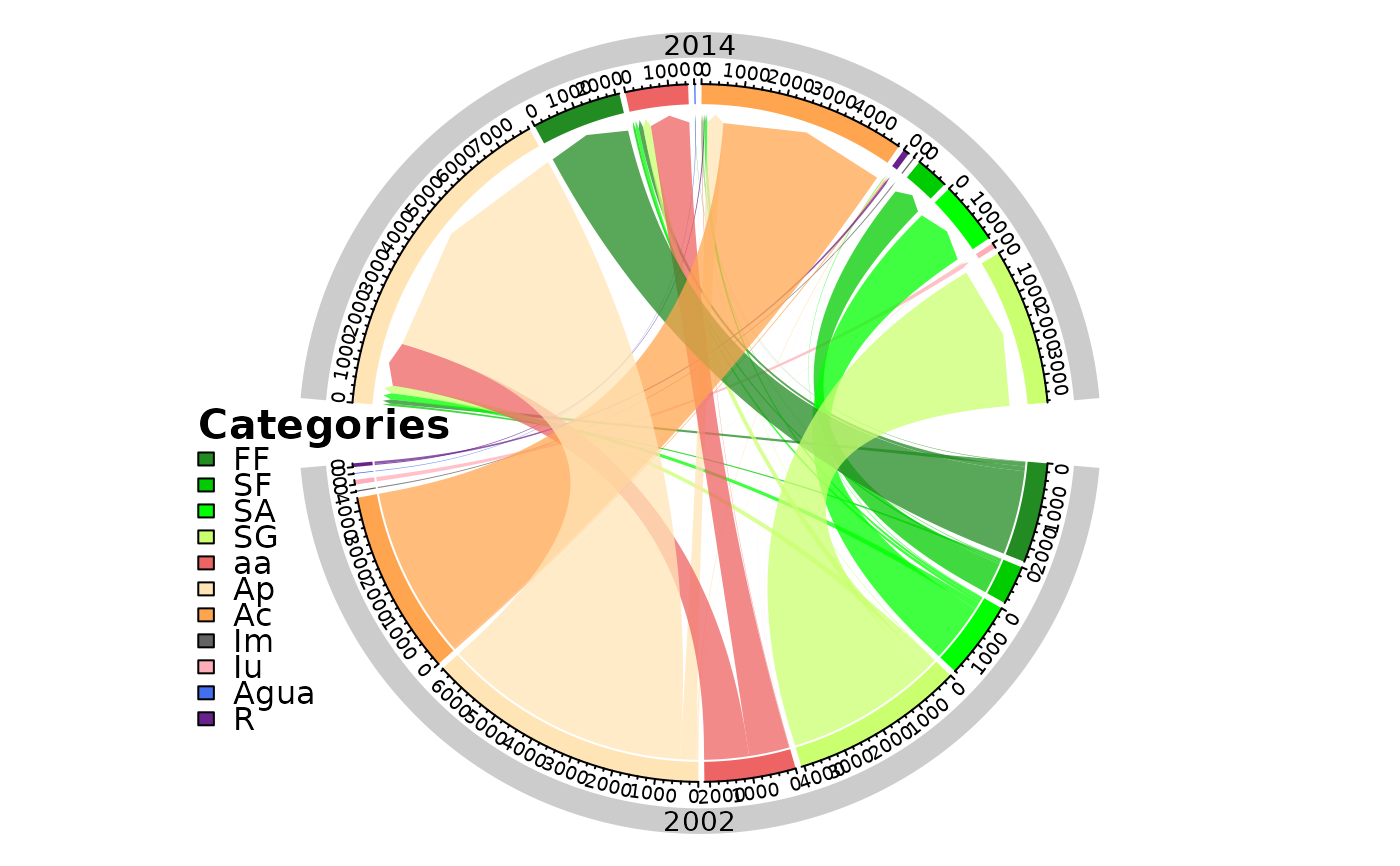A circlize plot representing the one step transitions between two times point of interest.

chordDiagramLand(
dataset,
legendtable,
legposition = c(x = -1.3, y = 0),
legtitle = "Categories",
sectorcol = "gray80",
area_km2 = TRUE,
legendsize = 1,
y.intersp = 1,
x.margin = c(-1, 1)
)

## Arguments

dataset A table of the one step transition (lulc_OneStep) generated by contingencyTable. A table containing the LUC legend items and their respective color (tb_legend). numeric. A vector containing the x and y values for the position of the legend. (see legend). character. The title of the legend. character. The color of the external sector containing the years of compared time points. logical. If TRUE the change is computed in km2, if FALSE in pixel counts. numeric. Font size of the legend. (see "cex" in legend). numeric. character interspacing factor for vertical (y) spacing in the legend. numeric vector ensuring additional space (blank area) on the left or right of the circle for the legend, by default it is c(-1, 1). (see "canvas.xlim" in circos.par)

A Chord Diagram

## Examples


# editing the category names

SL_2002_2014$tb_legend$categoryName <- factor(c("Ap", "FF", "SA", "SG", "aa", "SF",
"Agua", "Iu", "Ac", "R", "Im"),
levels = c("FF", "SF", "SA", "SG", "aa", "Ap",
"Ac", "Im", "Iu", "Agua", "R"))

SL_2002_2014$tb_legend$color <- c("#FFE4B5", "#228B22", "#00FF00", "#CAFF70",
"#EE6363", "#00CD00", "#436EEE", "#FFAEB9",
"#FFA54F", "#68228B", "#636363")

# the plot
chordDiagramLand(dataset = SL_2002_2014$lulc_Onestep, legendtable = SL_2002_2014$tb_legend)